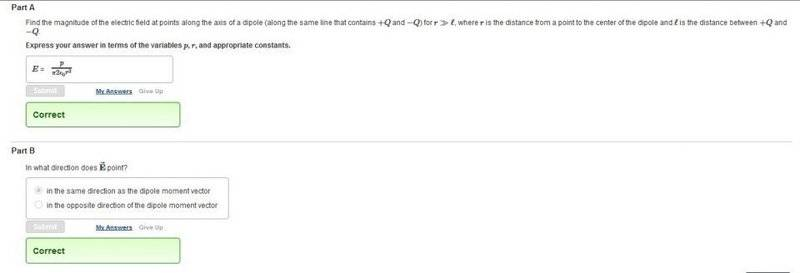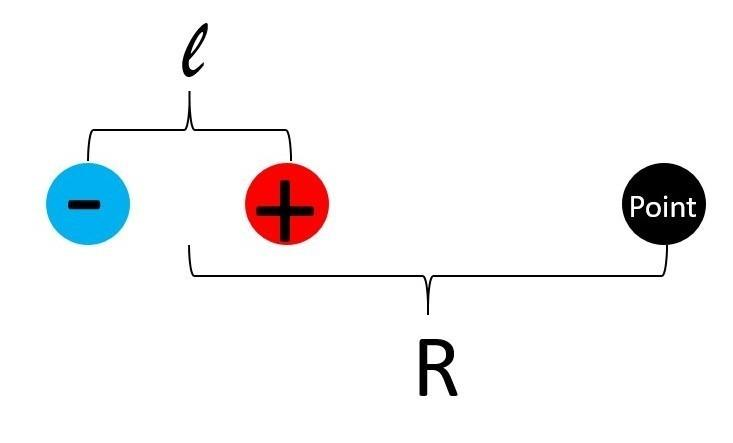# Dipole magnitude and direction (21.67)

## Homework Statement## Homework Equations

Total electric field = Electric field caused by Q_1 + electric field caused by Q_2
##\vec{e} = \vec{e_1}+\vec{e_2}##
Dipole moment = ##P = Ql##

## The Attempt at a Solution**For Part A**
##\vec{E} = \vec{E_1}+\vec{E_2}##
The charges are opposite so it will be a difference, not a sum.
##\frac{KQ}{(R+\frac{l}{2})^2} - \frac{KQ}{(R-\frac{l}{2})^2}##
Define ##A=(R+\frac{l}{2})^2 ## and ##B=(R-\frac{l}{2})^2##
Then ##\frac{KQ}{A} - \frac{KQ}{B}##
##\frac{BKQ-KQA}{AB}##
##\vec{E}=\frac{KQ(B-A)}{AB}##
After simplification, ##AB=R^4 + \frac {(R^2) (l^2)}{2}-R^2 l^2 + \frac{l^4}{4}## and ##B-A=-2Rl##
I didn't get the answer above in part A. Maybe my algebra is wrong or something else?

**Now for Part B**
I feel that ##\vec{E}## should be in the opposite direction of the dipole moment vector (DMP). In the picture above, if the neg charge is on the left and the pos on the right, then the dmp points to the left, but the field at the point points to the right, is this correct? Something similar will happen if we switch the positions of the two charges?

THANK you very much!

BvU
Homework Helper
Must say I can't understand the problem statement. You have the correct answers in front of you ?

I didn't get the answer above in part A
For R >> l you can ignore terms like R2l2 aside R4.

if the neg charge is on the left and the pos on the right, then the dmp points to the left
No, it points to the right.

•Calpalned
No, it points to the right.
Yes you are right. I read the textbook and the Dipole Moment points from the neg charge to the positive one.
So I understand part B. But what about part A?

BvU